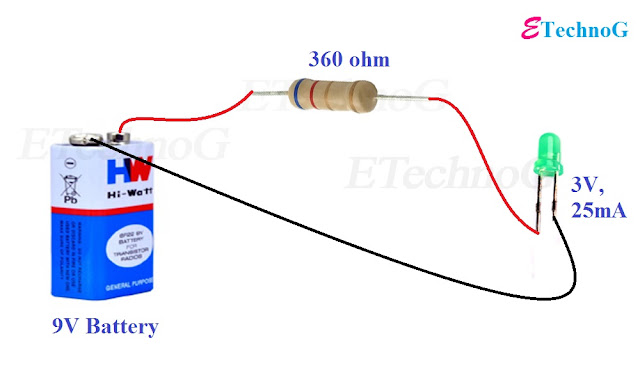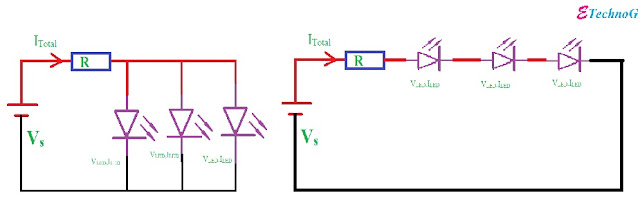## Resistor value Calculation for LED

Today we are going to learn how to calculate the value of resistor for LED when connecting with battery or any DC source.
If you see the picture carefully then you will understand How to calculate the value of the resistor for LED when connecting with battery or any DC source.

### How to calculate the value of the resistor for LED when connecting with Power Supply?

Now let's known how to calculate the value of resistor for LED when connecting with battery or any DC source.
The equation for calculating the value of the resistor is, (Vsupply - Vled)/Iled

So at first find the value of the supply voltage, LED rating Voltage, LED rating Current. Then put the values on the above equation and calculate the value of the resistor.
If you did not get the resistor which is the result of the calculation in the market then you can take the resistor of rating more than your calculated value which is available in the market.Resistor value Calculation for LED.

Example:

For example, in the below picture we have taken a 9V battery and a LED of 3V,25mA. The required resistor is 360ohm which is connected in series with the LED.Example of Resistor value Calculation for LED.

### The connection of more than One LEDs in Series and Parallel:

Now if you want to connect more than one LED then, What You Do?
If you connect the LEDs in series then take the value of Current(Iled) of anyone LED and Take the value of Voltage(Vled) of the summation of the total no of LEDs.

For Example, if you want to connect the five LEDs in series of  3V,25mA rating then take the value of Current(Iled)= 25mA and Voltage(Vled)= (3+3+3+3+3)=15V.LEDs in series and parallel.

Now if you connect the LEDs in parallel then take the value of Current(Iled) of summation of total no of LED and Voltage(Vled) of anyone LED.

For Example, if you want to connect the five LEDs in parallel of  3V,25mA rating then take the value of Current(Iled)=(25+25+25+25+25)mA=125mA and Voltage(Vled)=3V.

### What You Do? If you do not know the current rating of the LED?

Most of the cases we have known the voltage rating of the LED but we do not know the Current rating of the LED. In this cases first, measure the resistance across the LED then put the value on the equation Iled=Vled/Rled and calculate the current of the LED.

### How to calculate the power rating of the Resistor?

It is very simple. The equation for calculating the power rating of the resistor is P= (Vsupply - Vled)*Iledwatt

How to calculate the value of resistor for LED when connecting with Power Supply.Reviewed by Author on January 09, 2019 Rating: 5